### Home > MC1 > Chapter 4 > Lesson 4.3.4 > Problem4-120

4-120.

Copy the following number line on your paper. Then place each number below on the line in its appropriate place.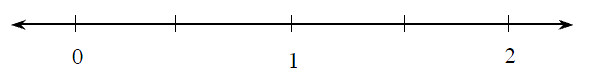1.  $\frac { 70 } { 100 }$

1.  $118\%$

1.  $0.085$

1.  $1.60$

1.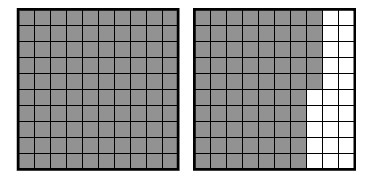Think about how big each number is. It may help to change each number to a percent.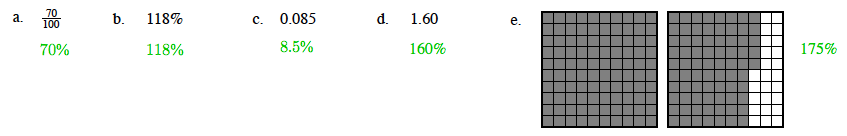Next, try placing the numbers greater than $1$ (or $100\%$) on the number line in their appropriate places.
We can write the numbers greater than one in order from smallest to largest: $118\%\ \ 160\%\ \ 175\%$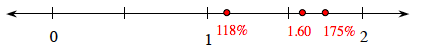Now compare the numbers less than one ($100\%$) and place them on the number line.
Note that $8.5\%$ is the smallest number and $70\%$ is the next. $8.5\%\ \ 70\%$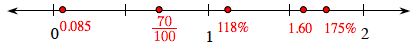The number line is now complete.